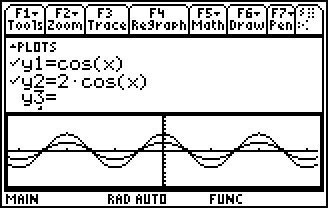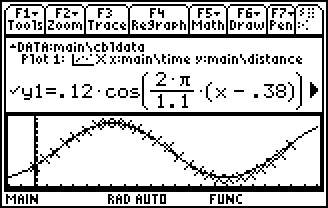# Activities

••• ##### Subject Area

• Math: Calculus: Transcendental Functions
• Math: Calculus: Applications of the Derivative
• Math: Calculus: Derivatives

• ##### Author9-12

45 Minutes

• ##### Device
• TI-89 / TI-89 Titanium

TI Connect™

CBR™/CBR 2™

• ##### Other Materials
pendulum (smooth ball hanging from a string), meter stick, stop watch

## Gettin' the Swing#### Activity Overview

Students investigate sinusoidal functions, collect data from a swinging pendulum, model the data with a cosine function, and take the derivative to find the velocity and acceleration.

#### Key Steps

•Students begin the activity by reviewing the range, period, and amplitude of a sinusoidal function. Students will then explore sinusoidal functions and the effect of the parameters A, B, C, and D in the equation y = Acos(B(xD)) + C.

•In Part 2, students connect a motion detector and easily begin collecting data of a swinging pendulum. Student will write a function which models the data and take the derivative to model the velocity and acceleration. Students can verify their solution with the velocity and acceleration data. Additional data is supplied for use as an extension or for students who don’t have access to a motion detector.